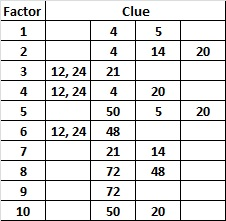# 10 Just Like Sudoku?

10 is a composite number. 10 = 1 x 10 or 2 x 5. Factors of 10: 1, 2, 5, 10. Prime factorization: 10 = 2 x 5.When 10 is a clue in the FIND THE FACTORS puzzles, either 1 x 10 or else 2 x 5 will work for that particular puzzle.

On numerous occasions when I have demonstrated how to solve a Find the Factors puzzle, someone will remark that the puzzle is just like Sudoku. What are common factors that both a Find the Factors puzzle and a Sudoku puzzle will have? 1) Both will have only one solution. 2) Both require the solver to be able to count, write, and place the numerals 1 to 9, but Find the Factors also requires the number 10 be placed. 3) Both were originally designed to require logic to be solved. 4) Both puzzles utilize a square grid. 5) Both puzzles have several difficulty levels and variations that make the puzzles more challenging.

What factors do the two puzzles NOT have in common? 1) A difficult Sudoku puzzle can take some people almost an hour to solve while Find the Factors  would never take that long. 2) Sudoku has been a wildly popular puzzle while Find the Factors is known only among a small circle of people who have had some kind of contact with me. 3) Some more recent Sudoku puzzles require the solvers to guess and check which is getting away from its logic puzzle roots and is making it less popular for some people.  4) Sudoku could just as easily be made with letters of an alphabet, colors, or the names of the planets (if you include Pluto), while Find the Factors has to be made with numbers. 5) Sudoku requires only counting, while Find the Factors also requires the solver to factor and multiply. So really, if Find the Factors were just like Sudoku, it would look like this:Requiring skip counting to solve the Skipoku puzzle does make it more challenging, but I became annoyed with the skip counting by the time I finished the puzzle.

One complaint about some advanced Sudoku puzzles is the need to guess and check to find a solution. Is it necessary to guess and check all the possibilities to solve this level SIX Find the Factors puzzle?Click 10 Factors 2013-11-25 for more puzzles.

No. Guessing and checking is not necessary even though the clues 12 and 24 have several common factors.  We easily eliminate 1 and 2 because both of them would require a partner greater than 10. We also eliminate 12 because it is greater than 10. What about 3, 4, and 6? Do we have to try each of those possibilities? When we examine the puzzle we notice that it has 10 clues with only two of the clues paired together. We also notice there is one column that contains no clue, so supposedly any factor could fit there. Here is a chart of all the possible factors and the clues each could satisfy.Remember that each factor must be written twice, once in the factor row and one in the factor column. Notice that the number 9 is a factor of only one of the clues. That means that 9 has to be put over the column with no clues. From there it is easy to know where the other 9 goes and both 8’s and so forth until it is completed. Not all level SIX puzzles can be completed that easily, but using logic instead of guessing and checking is the key to solving these puzzles.

# 3 Puzzles for a November Day

3 is the smallest odd prime number.

3 is the only number that equals the sum of ALL the counting numbers less than itself.

• 3 is a prime number.
• Prime factorization: 3 is prime.
• The exponent of prime number 3 is 1. Adding 1 to that exponent we get (1 + 1) = 2. Therefore 3 has exactly 2 factors.
• Factors of 3: 1, 3
• Factor pairs: 3 = 1 x 3
• 3 has no square factors that allow its square root to be simplified. √3 ≈ 1.732.How do we know that 3 is a prime number? If 3 were not a prime number, then it would be divisible by at least one prime number less than or equal to √3 ≈ 1.7. Since there are no prime numbers less than or equal to 1.7, we know 3 is a prime number.

When 3 is a clue in the FIND THE FACTOR puzzles, the factors are always 1 and 3.

—————————————————————————————

Click on this link, 10 factors 2013-11-04, to get printable copies of the puzzles below and last Monday’s answers. To solve the puzzles, place the numbers 1 – 10 in both the top row and the first column so that those numbers are the factors of the given clues. Obviously level 6 is more difficult to solve than level 1.